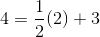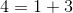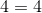# PSAT Math : How to find out if a point is on a line with an equation

## Example Questions

### Example Question #477 : Sat Mathematics

In the xy -plane, line is given by the equation 2x - 3y = 5. If line passes through the point (a ,1), what is the value of a ?

-2
5
4
-1
3
Explanation:

The equation of line l relates x -values and y -values that lie along the line. The question is asking for the x -value of a point on the line whose y -value is 1, so we are looking for the x -value on the line when the y-value is 1. In the equation of the line, plug 1 in for and solve for x:

2x - 3(1) = 5

2x - 3 = 5

2x = 8

x = 4. So the missing x-value on line l is 4.

### Example Question #478 : Sat Mathematics

The equation of a line is: 2x + 9y = 71

Which of these points is on that line?

(4,-7)

(2,7)

(-2,7)

(-4,7)

(4,7)

(4,7)

Explanation:

Test the difference combinations out starting with the most repeated number.  In this case, y = 7 appears most often in the answers.  Plug in y=7 and solve for x.  If the answer does not appear on the list, solve for the next most common coordinate.

2(x) + 9(7) = 71

2x + 63 = 71

2x = 8

x = 4

Therefore the answer is (4, 7)

### Example Question #1 : Points And Distance Formula

Which of the following lines contains the point (8, 9)?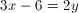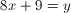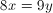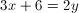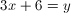Explanation:

In order to find out which of these lines is correct, we simply plug in the values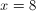and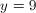into each equation and see if it balances.

The only one for which this will work is### Example Question #1 : How To Find Out If A Point Is On A Line With An Equation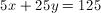Which point lies on this line?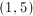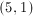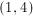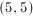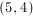Explanation:Test the coordinates to find the ordered pair that makes the equation of the line true: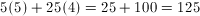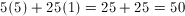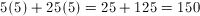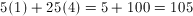### Example Question #1 : How To Find Out If A Point Is On A Line With An Equation

Points D and E lie on the same line and have the coordinates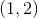and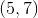, respectively.  Which of the following points lies on the same line as points D and E?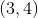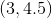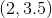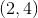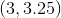Explanation:

The first step is to find the equation of the line that the original points, D and E, are on.  You have two points, so you can figure out the slope of the line by plugging the points into the equation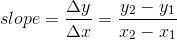.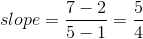Therefore, you can get an equation in the line in point-slope form, which is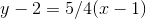.

Plug in the answer options, and you will find that only the pointsolves the equation.

### Example Question #2 : How To Find Out If A Point Is On A Line With An Equation

Which of the following points is on the line given by the equation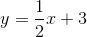?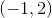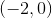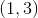Explanation:

In order to solve this, try each of the answer choices in the equation:For example, when we try (3,4), we find: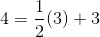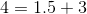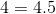This does not work. When we try all the choices, we find that only (2,4) works: